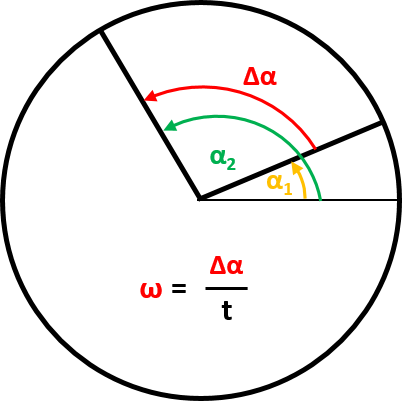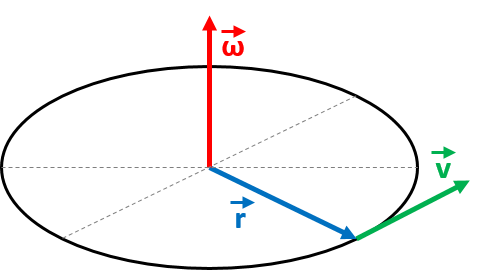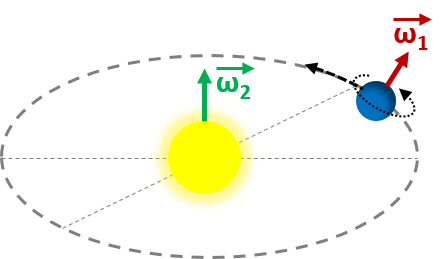# Angular Velocity Calculator

Created by Wojciech Sas, PhD candidate
Reviewed by Bogna Szyk and Jack Bowater
Last updated: Dec 15, 2021

This angular velocity calculator is a simple-to-use tool that gives an immediate answer to the question "How to find angular velocity?". In the text you'll find several angular velocity formulas, learn about different angular velocity units, and, finally, estimate the angular velocity of the Earth! Have you ever wondered what is the relation between angular velocity and angular frequency, or where angular velocity is applied? Read on to find out, and become an expert in the field of circular motion.

## What is angular velocity?

Angular velocity describes the rotational movement of bodies. It measures how fast they move around some center of rotation. We can think about two different kinds of rotations. The first one describes the motion of the center of mass of a given object around a specific point in space, which can be described as an origin. Some examples include planets moving around the Sun, or a car taking an exit on the highway.

The second one tells about the rotation of the body around its own center of mass - the spin (not to be confused with the quantum property of particles, also called spin). Surely you've seen a basketball player spinning a ball on his finger.

Generally, we can say the faster the movement, the higher the angular velocity. To define some specific values, we have to move on to the angular velocity equations, described in the next section.

## Angular velocity formulasIn this angular velocity calculator, we use two different formulas of angular velocity, depending on what input parameters you have.

The first angular velocity equation is analogous to the equation for linear velocity:

ω = (α₂ - α₁) / t = Δα / t,

where α₁ and α₂ are two values of angles on a circle, and Δα is their difference. t is the time in which the angle change occurs. As you can see, for the normal velocity there is a ratio of the positional change in a period, while here we use angle instead of distance.The second angular velocity formula can be derived from the relation of the linear velocity and the radius using the cross product, which is:

v = ω × r.

We can rewrite this expression to obtain the equation of angular velocity:

ω = r × v / |r|²,

where all of these variables are vectors, and |r| denotes the absolute value of the radius. Actually, the angular velocity is a pseudovector, the direction of which is perpendicular to the plane of the rotational movement.

## Angular velocity units

There are multiple units of angular velocity, and the ones which are used in our angular velocity calculator are mentioned below:

• rad/s or radians per second - the definition which comes straight out of the first angular velocity formula. It tells how big the rotation (or angle) is that the body moves through in a given time,

• RPM or revolutions per minute - the unit which is found most frequently in practical application. Using it, you can describe how fast a wheel or an engine is spinning. You can easily imagine the difference between 10 and 100 RPM.

• Hz or hertz - the same units that are used for frequency, but rarely used in the context of angular velocity. It is in some way similar to RPM, telling us how many full rotations are made in a given time. The difference is that previously the basic unit of time was a minute, and here it is a second.

Naturally, all of these angular velocity units converted between one another using the following relations:

1 RMP = 0.10472 rad/s = 0.01667 Hz,

or the other way round:

1 Hz = 6.283 rad/s = 60 RPM.

## Angular velocity vs. angular frequency

Have a look at the definition of the angular frequency:

ω = 2 * π * f,

where f is the frequency. As we can see, it is denoted by the same letter. Additionally, the unit of angular frequency is rad/s, precisely the same as for the angular velocity. So the question may arise: "What is the difference between angular velocity and angular frequency?".

The answer is relatively simple. The relation between angular frequency and angular velocity is analogous to the one between the speed and the velocity. The first one is a magnitude of the latter, or in other words, angular frequency is a scalar, whereas angular velocity is a (pseudo)vector.

The angular frequency is commonly used when talking about a harmonic motion, an example of which is a simple pendulum. As you can imagine, the motion doesn't have to be represented by a standard rotation, but just a movement which periodically repeats its position. The angular velocity, however, is strictly connected with the motion around some point. Therefore, we can say that angular frequency is a more general quantity and can be used to describe a wide range of physical problems, while the angular velocity includes only rotational movement.

🙋 We have a dedicated tool that explains how to calculate angular frequency. Be sure to check it too!

## How to find angular velocity of the Earth?

How about we make use of our angular velocity calculator? Let's estimate the angular velocity of the Earth! Firstly, we consider the spinning velocity. We know that the Earth is making a full rotation, with respect to distant stars, in about 23 h 56 min 4 s, which is roughly 23.934 h. The full rotation is the angle 2π rad, so the resulting angular velocity is:

ω₁ = 2π rad / 23.934 h = 0.2625 rad/h = 0.00007292 rad/s,

or 7.292 * 10⁻⁵ rad/s (using scientific notation).

Now that we know the spin angular velocity of the Earth, we can evaluate its linear velocity at the equator. To do so, we need the radius of the Earth, which is roughly 6,371 km. The only thing we have to do is to insert values into the second formula of angular velocity:

v₁ = r₁ * ω₁ = 6,371 km * 7.292 * 10⁻⁵ rad/s = 0.4646 km/s = 464.6 m/s.

To work out the linear velocity with respect to the center of the Earth, all you need to do is to multiply this result by the cosine of the latitude of your city. By the way, have you ever wondered why rockets usually launch from spaceports placed near the equator and not from the poles? Well, almost 500 m/s boost at the beginning is the considerable fraction of its final velocity. So moving the start point as close to the equator as possible reduces the amount of fuel needed to accelerate the rocket.After that, we can ask once again how to find the angular velocity of Earth, but this time the orbital one. All the calculations are analogical, but we have to change the time from 23.943 h to one year, which is about 365.25 days. The angle change is the same, the complete revolution.

ω₂ = 2π rad / 23.934 h = 0.0000001991 rad/s = 1.991 * 10⁻⁷ rad/s,

and the linear velocity of the Earth with respect to the Sun (for the average radius 1.496 * 10⁸ km) is:

v₂ = 1.496 * 10⁸ km * 1.991 * 10⁻⁷ rad/s = 29.785 km/s.

We are moving pretty fast, aren't we?

## Physical quantities dependent on the angular velocity

There are numerous physical quantities which are related to angular velocity, some of which are listed below:

1. The angular acceleration - describes how the angular velocity changes with time. The higher the difference of angular velocities, the bigger the value of angular acceleration.

2. The rotational kinetic energy - the measure of energy in circular motion. As with kinetic energy, the dependency of (angular) velocity is quadratic.

3. The centrifugal force - it can be felt in a vehicle when it turns. The faster you take a turn, or the sharper it is, the higher the centrifugal force gets, which can be clearly felt.

4. The Coriolis effect - which causes objects to turn, if they are placed on a rotating body (e.g. on the Earth), instead of moving in a straight line.

5. The system of pulleys - this is not a physical quantity, precisely speaking, but it is an interesting device which is all about angular velocity. The simplest system consists of two pulleys, usually with different circumferences or radii. They are connected by a belt, so their linear velocities are identical, but since they are of different sizes, their angular velocities change proportionally. Knowing this and having some engine with well defined rotational velocity, we can set the angular velocity of the output element with good precision, simply by adjusting its size.

## Conservation of angular momentum

There are a few fundamental rules which tell us about the quantities conserved in isolated systems. The best-known are the conservation of energy and the conservation of momentum. Together with them, there is also the conservation of angular momentum. If we think of two moments in time, the rule can be written as:

I₁ * ω₁ = I₂ * ω₂,

where I₁ and I₂ are the initial and the final mass moments of inertia respectively, the quantities which describe the distribution of mass with respect to the center of the bodies.

We can see that if the moment of inertia increases, the angular velocity decreases, and vice versa. So, what are the consequences of this phenomenon? Let's imagine that you are a figure skater. When you rotate, you possess some angular velocity. If your arms are wide open, the mass moment of inertia is relatively big. Then, you move arms close to the rest of the body. As a consequence, your moment of inertia decreases, so due to the fact the overall angular momentum has to be conserved, your angular velocity increases - it means you will spin faster! This is no magic, just physics!

If you can't don't like skating, you can try to verify the rule with a usual swivel chair. Just remember, safety first! Make sure there is enough space to do this experiment. After that, simply start spinning and see how your angular velocity changes when you move your arms back and forth. Additionally, you can increase the effect by using some dumbbells. As a result, you can combine both exercising and fun into one thing!

Wojciech Sas, PhD candidate
#1 Angle difference
Angle change (Δα)
deg
Time (t)
sec
Angular velocity (ω)
Velocity (v)
m/s
m
Angular velocity (ω)
People also viewed…

### Coffee kick

A long night of studying? Or maybe you're on a deadline? The coffee kick calculator will tell you when and how much caffeine you need to stay alert after not sleeping enough 😀☕ Check out the graph below!

### Discount

Discount calculator uses a product's original price and discount percentage to find the final price and the amount you save.

### Malus law

With this Malus law calculator you can find the intensity of light which passes through the polarizer taking into account the direction of its initial polarization.

### Physical Pendulum

The Physical Pendulum Calculator helps you compute the period and frequency of a physical pendulum.